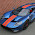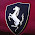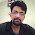### C Program to add two polynomials using Linked list..

#include<stdio.h>
#include<stdlib.h>

struct node
{
int coef,expo;
struct node* next;
};

struct node* insertpoly(struct node* thead,int c,int e);
struct node* append(struct node* thead,int c,int e);
struct node* polyaddition(struct node* p1thead,struct node* p2thead);
void display(struct node* thead);

void main()
{
int a,b,n,i;
struct node* p1head,* p2head,* p3head;
p1head=p2head=NULL;

// Inputing the first polynomial..

printf("Enter the no of terms of polynomial 1..");
scanf("%d",&n);
printf("\nEnter the polynomial..");
for(i=0;i<n;i++){
printf("\nEnter the coefficient and exponent of the term..");
scanf("%d%d",&a,&b);
p1head=insertpoly(p1head,a,b);
}

// Inputing the second polynomial..

printf("\nEnter the no of terms of polynomial 2..");
scanf("%d",&n);
printf("\nEnter the polynomial..");
for(i=0;i<n;i++){
printf("\nEnter the coefficient and exponent of the term..");
scanf("%d%d",&a,&b);
p2head=insertpoly(p2head,a,b);
}

//Performing Addition..

p3head=polyaddition(p1head,p2head);

//Displaying the polynomial..

printf("\nThe polynomial 1 is..");
display(p1head);
printf("\nThe polynomial 2 is..");
display(p2head);
printf("\nThe sum of the two polynomials is..");
display(p3head);
}

struct node* append(struct node* thead,int c,int e)
{
struct node* newnode = (struct node*)malloc(sizeof(struct node));
newnode->coef=c;
newnode->expo=e;
if(thead==NULL){// Corner Case to handle if the list is empty...
newnode->next=NULL;
return newnode;
}
struct node* trav=thead;
while(trav->next!=NULL) // Traversing to point to the last node...
trav=trav->next;
trav->next=newnode;
newnode->next=NULL;
return thead;
}

struct node* insertpoly(struct node* thead,int c,int e)
{
struct node* newnode=(struct node*)malloc(sizeof(struct node));
newnode->coef=c;
newnode->expo=e;
if(thead==NULL){            // for inserting the first node..
newnode->next=NULL;
return newnode;
}
struct node* prev,* curr;
prev=curr=thead;
while(curr!=NULL && curr->expo>e){
prev=curr;
curr=curr->next;
}
if(curr==thead){            // for inserting before the first node...
newnode->next=curr;
return newnode;
}
else if(curr==NULL){        //for inserting after the last node....
prev->next=newnode;
newnode->next=NULL;
}
else{
newnode->next=curr;
prev->next=newnode;
}
return thead;
}

struct node* polyaddition(struct node* p1thead,struct node* p2thead)
{
struct node* ans=NULL;
struct node* t1,* t2;
t1=p1thead;
t2=p2thead;
while(t1!=NULL && t2!=NULL){
if(t1->expo > t2->expo){
ans=append(ans,t1->coef,t1->expo);
t1=t1->next;
}
else if(t1->expo < t2->expo){
ans=append(ans,t2->coef,t2->expo);
t2=t2->next;
}
else{
ans=append(ans,(t1->coef)+(t2->coef),t1->expo);
t1=t1->next;
t2=t2->next;
}
}

while(t1!=NULL){            //coping the remaining terms of polynomial 1...
ans=append(ans,t1->coef,t1->expo);
t1=t1->next;
}

while(t2!=NULL){            //coping the remaining terms of polynomial 2...
ans=append(ans,t2->coef,t2->expo);
t2=t2->next;
}
return ans;
}

void display(struct node* thead)
{
struct node* temp=thead;
if(temp==NULL){
printf("\nEmpty..");
}
else{
while(temp->next!=NULL){
printf(" %dx^%d +",temp->coef,temp->expo);
temp=temp->next;
}
printf(" %dx^%d ",temp->coef,temp->expo);
}
}

#### 8 comments:

1.This program is really very good.Here there is no drawbacks.It solve all the cases (i.e) even if the user enters unsorted polynomail

2.good program!!! It cleared my doubts

3.you are the best bro

4.attach subtract and multi operations to it

5.6.please attach subtract and multu to it

7.This comment has been removed by the author.

8.nice
https://ultratechbits.blogspot.com/2018/11/addition-of-two-polynomials-linked-list.html